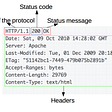# Building a SIEM: centralized logging of all Linux commands with ELK + auditd

`.*nmap.*`
`apt-get install auditd audispd-plugins`
`-a always,exit -F arch=b32 -S execve-a always,exit -F arch=b64 -S execve`
`systemctl enable auditd.servicesystemctl start auditd.service `
`auditctl -l`
`-a always,exit -F arch=b32 -S execve -F euid=0-a always,exit -F arch=b64 -S execve -F euid=0`
`type=SYSCALL msg=audit(1596148543.861:63): arch=c000003e syscall=59 success=yes exit=0 a0=565156eecbc0 a1=565156ef1fa0 a2=565156e97ce0 a3=8 items=2 ppid=1667 pid=2806 auid=1000 uid=0 gid=0 euid=0 suid=0 fsuid=0 egid=0 sgid=0 fsgid=0 tty=pts0 ses=1 comm=”whoami” exe=”/usr/bin/whoami” key=(null)type=EXECVE msg=audit(1596148543.861:63): argc=1 a0=”whoami”type=CWD msg=audit(1596148543.861:63): cwd=”/home/sec”type=PATH msg=audit(1596148543.861:63): item=0 name=”/usr/bin/whoami” inode=1185 dev=08:02 mode=0100755 ouid=0 ogid=0 rdev=00:00 nametype=NORMAL cap_fp=0000000000000000 cap_fi=0000000000000000 cap_fe=0 cap_fver=0type=PATH msg=audit(1596148543.861:63): item=1 name=”/lib64/ld-linux-x86–64.so.2" inode=131848 dev=08:02 mode=0100755 ouid=0 ogid=0 rdev=00:00 nametype=NORMAL cap_fp=0000000000000000 cap_fi=0000000000000000 cap_fe=0 cap_fver=0type=PROCTITLE msg=audit(1596148543.861:63): proctitle=”whoami”`
`ausearch -i -if /tmp/file`
`type=SYSCALL msg=audit(1596149019.479:174): arch=c000003e syscall=59 success=yes exit=0 a0=562e0acc36c0 a1=562e0ad523c0 a2=562e0ae1df70 a3=8 items=2 ppid=2879 pid=2903 auid=1000 uid=1000 gid=1000 euid=1000 suid=1000 fsuid=1000 egid=1000 sgid=1000 fsgid=1000 tty=pts2 ses=4 comm=”cat” exe=”/bin/cat” key=(null)type=EXECVE msg=audit(1596149019.479:174): argc=5 a0=”cat” a1=”test1" a2=”test2" a3=”test3" a4=”test4"`
`def filter(event)   # Getting full log event   full_log = event.get("full_log")   # Making sure its of type EXECVE   command = ""   if /type=EXECVE/.match(full_log) then       log_execve = full_log.split("type=EXECVE")       # Spliting values       log_execve_kv = Hash[log_execve.split(" ").map{|x| x.split("=",2)}]       log_execve_kv.each do |key, value|           # If the key equals a followed by 1 or 2 numbers           if /a\d+|a\d+\d+/.match(key) then               # Si value starts with "               if /"/.match(value) then                   command += value[1..-2] + " "               else                   # Spliting the value in a two by two array                   convert = [value].first.scan(/../)                   convert.each_with_index { |item, index|                   #Substituting 00 by 20                   if item == "00"                       convert[index] = 20                   end                   }                   outputJoin = convert.join()                   output = [outputJoin].pack('H*')                   command += output + " "               end           end       end   end   # Save the output in the [command] field   event.set("command", command.chop)   return [event]end`
`if “auditd” in [decoder][name] { ruby {path => “/etc/logstash/audit_command.rb”}}`

--

--

--

## More from Security Shenanigans

I’m a security engineer who enjoys writing about experiences in the infosec field. linkedin.com/in/federlago.

Love podcasts or audiobooks? Learn on the go with our new app.

## What is DNS? | How DNS works?## How Web Browsers work?## Manufacturers: Approach Cybersecurity Like Your Assembly Line## How to hide from the State’s Big Brother? Snowden’s method to use## The Key to Smart Home Success: Safety and Security — ReadWrite## Security Shenanigans

I’m a security engineer who enjoys writing about experiences in the infosec field. linkedin.com/in/federlago.

## What is Automated Content Discovery?## ‘Dirty Pipe’ Linux Kernel Privilege Escalation Vulnerability (CVE-2022–0847)## How DNS Works: Domain Hierarchy, Record Types, Common attacks, and more…## Things You Must Know For Vehicle Hacking: PART 2